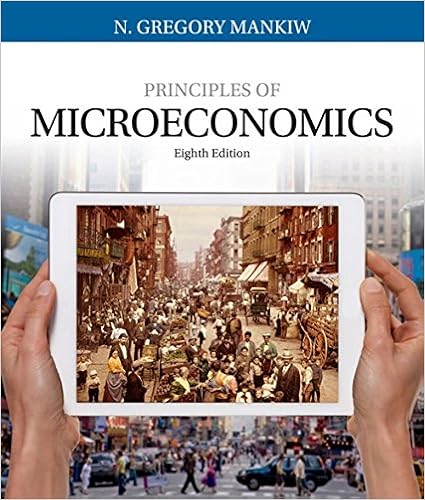# A recessionary expenditure gap is a the amount by

• Test Prep
• SkyB59
• 104
• 90% (97) 87 out of 97 people found this document helpful

This preview shows page 28 - 30 out of 104 pages.

##### We have textbook solutions for you!
The document you are viewing contains questions related to this textbook.The document you are viewing contains questions related to this textbook.
Chapter 9 / Exercise 3
Principles of Microeconomics
MankiwExpert Verified
182.A "recessionary expenditure gap" is: A. the amount by which the full-employment GDP exceeds equilibrium GDP.B.the amount by which aggregate expenditures fall short of those required to achieve the full-employment GDP.C. the amount by which investment exceeds saving at the full-employment GDP.D. the amount by which aggregate expenditures exceed the full-employment level of domestic output.
183.A recessionary expenditure gap exists if:
184.Refer to the above diagram. If the full-employment level of GDP is B and aggregate expenditures are at AE3, the:
185.Refer to the above diagram. If the full-employment level of GDP is B and aggregate expenditures are at AE3, the:
186.Refer to the above diagram. The value of the multiplier for this economy is: A. BC/hg.B. BC/AB.C. ed/di.D. df/BC.
187.Assume the current equilibrium level of income is \$200 billion as compared to the full-employment income level of \$240 billion. If the MPC is 0.6, what change in aggregate expenditures is needed to achieve full employment?
##### We have textbook solutions for you!
The document you are viewing contains questions related to this textbook.The document you are viewing contains questions related to this textbook.
Chapter 9 / Exercise 3
Principles of Microeconomics
MankiwExpert Verified
188.If the economy is in equilibrium at the \$400 billion level of GDP and the full-employment level of GDP is \$500 billion:
189.If the MPC is .50, all taxes are lump-sum taxes, and the equilibrium GDP is \$40 billion below the full-employment GDP, then the size of the recessionary expenditure gap:
•••